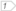Boost C++ Libraries

...one of the most highly regarded and expertly designed C++ library projects in the world.

tag

Metafunction defining type as the tag of the specified geometry type.

Description

With Boost.Geometry, tags are the driving force of the tag dispatching mechanism. The tag metafunction is therefore used in every free function.

Synopsis

template<typename Geometry>
struct tag
{
// ...
};

Template parameter(s)

Parameter

Description

typename Geometry

Any type fulfilling a Geometry Concept

Either

#include <boost/geometry.hpp>

Or

#include <boost/geometry/core/tag.hpp>

Metafunction result type

The metafunction tag defines type as one of the following tags:

• point_tag
• linestring_tag
• polygon_tag
• multi_point_tag
• multi_linestring_tag
• multi_polygon_tag
• box_tag
• segment_tag
• ring_tag

Compile time

Example

Shows how tag dispatching essentially works in Boost.Geometry

#include <iostream>

#include <boost/geometry.hpp>
#include <boost/geometry/geometries/polygon.hpp>
#include <boost/geometry/geometries/multi_polygon.hpp>

#include <boost/assign.hpp>BOOST_GEOMETRY_REGISTER_BOOST_TUPLE_CS(cs::cartesian)

template <typename Tag> struct dispatch {};

// Specialization for points
template <> struct dispatch<boost::geometry::point_tag>
{
template <typename Point>
static inline void apply(Point const& p)
{
// Use the Boost.Geometry free function "get"
// working on all supported point types
std::cout << "Hello POINT, you are located at: "
<< boost::geometry::get<0>(p) << ", "
<< boost::geometry::get<1>(p)
<< std::endl;
}
};

// Specialization for polygons
template <> struct dispatch<boost::geometry::polygon_tag>
{
template <typename Polygon>
static inline void apply(Polygon const& p)
{
// Use the Boost.Geometry manipulator "dsv"
// working on all supported geometries
std::cout << "Hello POLYGON, you look like: "
<< boost::geometry::dsv(p)
<< std::endl;
}
};

// Specialization for multipolygons
template <> struct dispatch<boost::geometry::multi_polygon_tag>
{
template <typename MultiPolygon>
static inline void apply(MultiPolygon const& m)
{
// Use the Boost.Range free function "size" because all
// multigeometries comply to Boost.Range
std::cout << "Hello MULTIPOLYGON, you contain: "
<< boost::size(m) << " polygon(s)"
<< std::endl;
}
};

template <typename Geometry>
inline void hello(Geometry const& geometry)
{
// Call the metafunction "tag" to dispatch, and call method (here "apply")
dispatch
<
typename boost::geometry::tag<Geometry>::type
>::apply(geometry);
}

int main()
{
// Define polygon type (here: based on a Boost.Tuple)
typedef boost::geometry::model::polygon<boost::tuple<int, int> > polygon_type;

// Declare and fill a polygon and a multipolygon
polygon_type poly;
boost::geometry::exterior_ring(poly) = boost::assign::tuple_list_of(0, 0)(0, 10)(10, 5)(0, 0);

boost::geometry::model::multi_polygon<polygon_type> multi;
multi.push_back(poly);

// Call "hello" for point, polygon, multipolygon
hello(boost::make_tuple(2, 3));
hello(poly);
hello(multi);

return 0;
}

 At the end to avoid conflicts with Boost.QVM

Output:

Hello POINT, you are located at: 2, 3
Hello POLYGON, you look like: (((0, 0), (0, 10), (10, 5), (0, 0)))
Hello MULTIPOLYGON, you contain: 1 polygon(s)